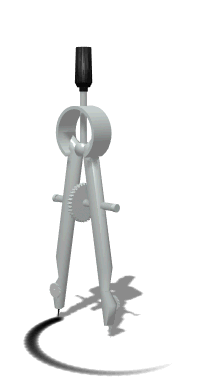•Math Policies & Procedures for Ms. Caswell's
7th and 8th Math
Classes
High Standards; High Achievement; High Expectations for AllMathematics is a subject that builds on concepts learned from the previous day.  Therefore, it is important to have practice and understanding of a concept before moving on to the next.  For this reason homework is assigned nearly every day.

# Classwork Practice (MATHia ®, Math Notes, Projects) = 10%

Homework (5 points daily)
Homework is graded each day and points are given according to whether or not the student has completed the assignment.  The full 5 points are given if the assignment is completed in pencil & on time, all steps are shown for each problem, and an answer is given. If homework is not complete, students have an opportunity to turn it in for late credit.  Late credit is worth a maximum of 70% (3.5 points).  If an assignment is not turned in, the student is still responsible for understanding the concepts in preparation for the test.  Homework is stamped daily.  Students should keep all homework until the end of each quarter.

If a student is absent, s/he has a one day grace period for EACH excused day of absence.  For example, if absent 2 days, the student is allowed to submit missed homework up to 2 days late for full credit.  It is the student’s responsibility to write down what they missed, do the assignments, and then bring them to me.

Tests (100 points) & Test Corrections/Retests

Assessment Tests are given at the end of each chapter.  A student earning less than a grade of 80% (B-) on a test is required to take a retest on a scheduled day.  In order to be prepared for the retest, a student must first complete test corrections for the original test.  Test corrections consist of writing out the incorrect problem, showing all work for calculating the new answer, and giving a written explanation of what was done incorrectly.  These completed test corrections are the student’s ticket to take the retest.  Students have several days to complete the test corrections and will be notified in advance when the retest day will be.  After every test, I send an email to the parents of students receiving less than 80% on the test.

MATHia® Software

We use a research-based software companion to the textbook called MATHia® one day per week in math class.  Students work through interactive math lessons customized specifically for their individual learning needs. The software is also available for students to use at home.  Students are highly encouraged to go on Mathia at least 2 hours per week outside of school.

Calculator use in Math Class

In both 7th & 8th grade math class, we use calculators almost every day because calculators help students learn mathematically complicated material and allow them to focus on the reasoning and problem solving ideas rather than on the act of calculating.  Researchers have studied classroom calculator use for several decades.  Rather than hampering mathematical ability, calculator use at the middle school level actually improves student math achievement including improvement of paper and pencil skills computation skills.  Please make sure your child has a calculator in his/her backpack at all times.

Supplies

In order to be prepared for math class each day, your child should have the following:
• Inexpensive but STURDY Calculator (not cell phone!)
• Headphones to keep in backpack (for MATHia® days)
• STURDY plastic folder with three prongs for math textbook
• Sharpened pencils & erasers (continuous supply!)
• Pen (any color)

Grades may be accessed at the Kyrene Website in ParentVue.   Grades are updated constantly, so please check your child’s progress in math frequently.

I’m looking forward to a fantastic year with your child!

Dondi Caswell